# cityseer.tools.graphs

Convenience functions for the preparation and conversion of networkX graphs to and from cityseer data structures.

Note that the cityseer network data structures can be created and manipulated directly, if so desired.

## snap_linestring_startpoint

snap_linestring_startpoint(
linestring_coords,
x_y)

Snaps a LineString’s start-point coordinate to a specified x_y coordinate.

### Parameters

linestring_coords
tuple | list | np.ndarray

A list, tuple, or numpy array of x, y coordinate tuples.

x_y
tuple[float, float]

A tuple of floats representing the target x, y coordinates against which to align the linestring start point.

### Returns

linestring_coords

A list of linestring coords aligned to the specified starting point.

## snap_linestring_endpoint

snap_linestring_endpoint(
linestring_coords,
x_y)

Snaps a LineString’s end-point coordinate to a specified x_y coordinate.

### Parameters

linestring_coords
tuple | list | np.ndarray

A list, tuple, or numpy array of x, y coordinate tuples.

x_y
tuple[float, float]

A tuple of floats representing the target x, y coordinates against which to align the linestring end point.

### Returns

linestring_coords

A list of linestring coords aligned to the specified ending point.

## align_linestring_coords

align_linestring_coords(
linestring_coords,
x_y,
reverse=False,
tolerance=0.5)

Align a LineString’s coordinate order to either start or end at a specified x_y coordinate within a given tolerance.

### Parameters

linestring_coords
tuple | list | np.ndarray

A list, tuple, or numpy array of x, y coordinate tuples.

x_y
tuple[float, float]

A tuple of floats representing the target x, y coordinates against which to align the linestring coords.

reverse
bool

If reverse=False the coordinate order will be aligned to start from the given x_y coordinate. If reverse=True the coordinate order will be aligned to end at the given x_y coordinate.

tolerance
float

Distance tolerance in metres for matching the x_y coordinate to the linestring_coords.

### Returns

linestring_coords

A list of linestring coords aligned to the specified endpoint.

names
routes
highways

## _EdgeInfo.__init__

_EdgeInfo()

Initialises a network information structure.

## _EdgeInfo.gather_edge_info

gather_edge_info(
edge_data)

Gather edge data from provided edge_data.

## _EdgeInfo.set_edge_info

set_edge_info(
nx_multigraph,
start_node_key,
end_node_key,
edge_idx)

Set accumulated edge data to specified graph and edge.

## nx_simple_geoms

nx_simple_geoms(
nx_multigraph,
simplify_dist=2)

Inferring geometries from node to node. Infers straight-lined geometries connecting the x and y coordinates of each node-pair. The resultant edge geometry will be stored to each edge’s geom attribute.

### Parameters

nx_multigraph
MultiGraph

A networkX MultiGraph with x and y node attributes.

simplify_dist
int

Simplification distance to use for simplifying the linestring geometries.

### Returns

MultiGraph

A networkX MultiGraph with shapely Linestring geometries assigned to the edge geom attributes.

## nx_from_osm

nx_from_osm(
osm_json)

Generate a NetworkX MultiGraph from Open Street Map data.

### Parameters

osm_json
str

A json string response from the OSM overpass API, consisting of nodes and ways.

### Returns

MultiGraph

A NetworkX MultiGraph with x and y attributes in WGS84 lng, lat geographic coordinates.

## nx_epsg_conversion

nx_epsg_conversion(
nx_multigraph,
from_epsg_code,
to_epsg_code)

Convert a graph from the from_epsg_code EPSG CRS to the to_epsg_code EPSG CRS. The to_epsg_code must be for a projected CRS. If edge geom attributes are found, the associated LineString geometries will also be converted.

### Parameters

nx_multigraph
MultiGraph

A networkX MultiGraph with x and y node attributes in the from_epsg_code coordinate system. Optional geom edge attributes containing LineString geoms to be converted.

from_epsg_code
int

An integer representing a valid EPSG code specifying the CRS from which the graph must be converted. For example, 4326 if converting data from an OpenStreetMap response.

to_epsg_code
int

An integer representing a valid EPSG code specifying the CRS into which the graph must be projected. For example, 27700 if converting to British National Grid.

### Returns

MultiGraph

A networkX MultiGraph with x and y node attributes converted to the specified to_epsg_code coordinate system. Edge geom attributes will also be converted if found.

## nx_wgs_to_utm

nx_wgs_to_utm(
nx_multigraph,
force_zone_number)

Convert a graph from WGS84 geographic coordinates to UTM projected coordinates. Converts x and y node attributes from WGS84 lng, lat geographic coordinates to the local UTM projected coordinate system. If edge geom attributes are found, the associated LineString geometries will also be converted. The UTM zone derived from the first processed node will be used for the conversion of all other nodes and geometries contained in the graph. This ensures consistent behaviour in cases where a graph spans a UTM boundary.

### Parameters

nx_multigraph
MultiGraph

A networkX MultiGraph with x and y node attributes in the WGS84 coordinate system. Optional geom edge attributes containing LineString geoms to be converted.

force_zone_number
int

An optional UTM zone number for coercing all conversions to an explicit UTM zone. Use with caution: mismatched UTM zones may introduce substantial distortions in the results.

### Returns

MultiGraph

A networkX MultiGraph with x and y node attributes converted to the local UTM coordinate system. If edge geom attributes are present, these will also be converted.

## nx_remove_filler_nodes

nx_remove_filler_nodes(
nx_multigraph)

Remove nodes of degree=2. Nodes of degree=2 represent no route-choice options other than traversal to the next edge. These are frequently found on network topologies as a means of describing roadway geometry, but are meaningless from a network topology point of view. This method will find and deleted these nodes, and replaces the two edges on either side with a new spliced edge. The new edge’s geom attribute will retain the geometric properties of the original edges.

Filler nodes may be prevalent in poor quality datasets, or in situations where curved roadways have been represented through the addition of nodes to describe arced geometries. cityseer uses shapely Linestrings to describe arbitrary road geometries without the need for filler nodes. Filler nodes can therefore be removed, thus reducing side-effects as a function of varied node intensities when computing network centralities.

### Parameters

nx_multigraph
MultiGraph

A networkX MultiGraph in a projected coordinate system, containing x and y node attributes, and geom edge attributes containing LineString geoms.

### Returns

MultiGraph

A networkX MultiGraph with nodes of degree=2 removed. Adjacent edges will be combined into a unified new edge with associated geom attributes spliced together.

## nx_remove_dangling_nodes

nx_remove_dangling_nodes(
nx_multigraph,
despine,
remove_disconnected=True,
cleanup_filler_nodes=True)

Remove disconnected components and optionally removes short dead-end street stubs.

### Parameters

nx_multigraph
MultiGraph

A networkX MultiGraph in a projected coordinate system, containing x and y node attributes, and geom edge attributes containing LineString geoms.

despine
bool

The maximum cutoff distance for removal of dead-ends. Use None or 0 where no despining should occur. Defaults to None.

remove_disconnected
bool

Whether to remove disconnected components. If set to True, only the largest connected component will be returned. Defaults to True.

cleanup_filler_nodes
bool

Removal of dangling nodes can result in “filler nodes” of degree two where dangling streets were removed. If cleanup_filler_nodes is True then these will be removed.

### Returns

MultiGraph

A networkX MultiGraph with disconnected components optionally removed, and dead-ends removed where less than the despine parameter distance.

## merge_parallel_edges

merge_parallel_edges(
nx_multigraph,
merge_edges_by_midline,
contains_buffer_dist)

Check a MultiGraph for duplicate edges; which, if found, will be merged. The shortest of these parallel edges is selected and buffered by contains_buffer_dist. If this buffer contains an adjacent edge, then the adjacent edge is merged. Edges falling outside this buffer are retained.

When candidate edges are found for merging, they are replaced by a single new edge. The new geometry selected from either:

• An imaginary centreline of the combined edges if merge_edges_by_midline is set to True;
• Else, the shortest edge is retained, with longer edges discarded.

### Parameters

nx_multigraph
MultiGraph

A networkX MultiGraph in a projected coordinate system, containing x and y node attributes, and geom edge attributes containing LineString geoms.

merge_edges_by_midline
bool

Whether to merge parallel edges by an imaginary centreline. If set to False, then the shortest edge will be retained as the new geometry and the longer edges will be discarded. Defaults to True.

contains_buffer_dist
int

The buffer distance to consider when checking if parallel edges are sufficiently similar to be merged.

### Returns

MultiGraph

A networkX MultiGraph with consolidated nodes.

## nx_iron_edges

nx_iron_edges(
nx_multigraph,
simplify=True,
simplify_dist=2,
straighten=True,
min_straightness_ratio=0.9975,
remove_wonky=True,
max_wonky_ratio=0.7,
wonky_dist_buffer=50)

Flattens edges straighter than min_straightness_ratio.

### Parameters

nx_multigraph
MultiGraph

A networkX MultiGraph in a projected coordinate system, containing x and y node attributes, and geom edge attributes containing LineString geoms.

simplify
bool

Whether to simplify the street geometries per simplify_dist.

simplify_dist
int

Ignored if simplify is False. Simplification distance to use for simplifying the linestring geometries.

straighten
bool

Whether to straighten edges where the ratio of the distance from a street’s start to end point divided by its length is greater than min_straightness_ratio.

min_straightness_ratio
float

Ignored if straighten is False. Edges with straightness greater than min_straightness_ratio will be flattened.

remove_wonky
bool

Straighten kinked street endings. This is intended for handling jagged endpoints arising from node consolidation processes.

max_wonky_ratio
float

Ignored if remove_wonky is False. The maximum straightness ratio to consider when looking for potentially wonky edges.

wonky_dist_buffer
int

Ignored if remove_wonky is False. The maximum distance to be searched from either end for wonky endpoints.

### Returns

MultiGraph

A networkX MultiGraph.

## nx_consolidate_nodes

nx_consolidate_nodes(
nx_multigraph,
buffer_dist=5,
min_node_group=2,
min_node_degree=1,
min_cumulative_degree,
max_cumulative_degree,
neighbour_policy,
crawl=False,
cent_min_degree=3,
cent_min_names,
cent_min_len_factor,
merge_edges_by_midline=True,
contains_buffer_dist=20)

Consolidates nodes if they are within a buffer distance of each other. Several parameters provide more control over the conditions used for deciding whether or not to merge nodes. The algorithm proceeds in two steps:

Nodes within the buffer distance of each other are merged. A new centroid will be determined and all existing edge endpoints will be updated accordingly. The new centroid for the merged nodes can be based on:

• The centroid of the node group;
• Else, all nodes of degree greater or equal to cent_min_degree;
• Else, all nodes with aggregate adjacent edge lengths greater than a factor of cent_min_len_factor of the node with the greatest aggregate length for adjacent edges.

The merging of nodes can create parallel edges with mutually shared nodes on either side. These edges are replaced by a single new edge, with the new geometry selected from either:

• An imaginary centreline of the combined edges if merge_edges_by_midline is set to True;
• Else, the shortest edge, with longer edges discarded; See merge_parallel_edges for more information.

### Parameters

nx_multigraph
MultiGraph

A networkX MultiGraph in a projected coordinate system, containing x and y node attributes, and geom edge attributes containing LineString geoms.

buffer_dist
float

The buffer distance to be used for consolidating nearby nodes. Defaults to 5.

min_node_group
int

The minimum number of nodes to consider a valid group for consolidation. Defaults to 2.

min_node_degree
int

The least number of edges a node should have in order to be considered for consolidation. Defaults to 1.

min_cumulative_degree
int

An optional minimum cumulative degree to consider a valid node group for consolidation. Defaults to None.

max_cumulative_degree
int

An optional maximum cumulative degree to consider a valid node group for consolidation. Defaults to None.

neighbour_policy
str

Whether all nodes within the buffer distance are merged, or only “direct” or “indirect” neighbours. Defaults to None.

crawl
bool

Whether the algorithm will recursively explore neighbours of neighbours if those neighbours are within the buffer distance from the prior node. Defaults to True.

cent_min_degree
int

The minimum node degree for a node to be considered when calculating the new centroid for the merged node cluster. Defaults to 3.

cent_min_names
int

The minimum number of cumulative street names or street references to be considered when calculating the new centroid. Requires names and routes edge attributes containing lists of OSM street names or route identifiers. Defaults to None.

cent_min_len_factor
float

The minimum aggregate adjacent edge lengths an existing node should have to be considered when calculating the centroid for the new node cluster. Expressed as a factor of the node with the greatest aggregate adjacent edge lengths. Defaults to None.

merge_edges_by_midline
bool

Whether to merge parallel edges by an imaginary centreline. If set to False, then the shortest edge will be retained as the new geometry and the longer edges will be discarded. Defaults to True.

contains_buffer_dist
int

The buffer distance to consider when checking if parallel edges are sufficiently similar to be merged.

### Returns

MultiGraph

A networkX MultiGraph with consolidated nodes.

### Notes

See the guide on graph cleaning for more information.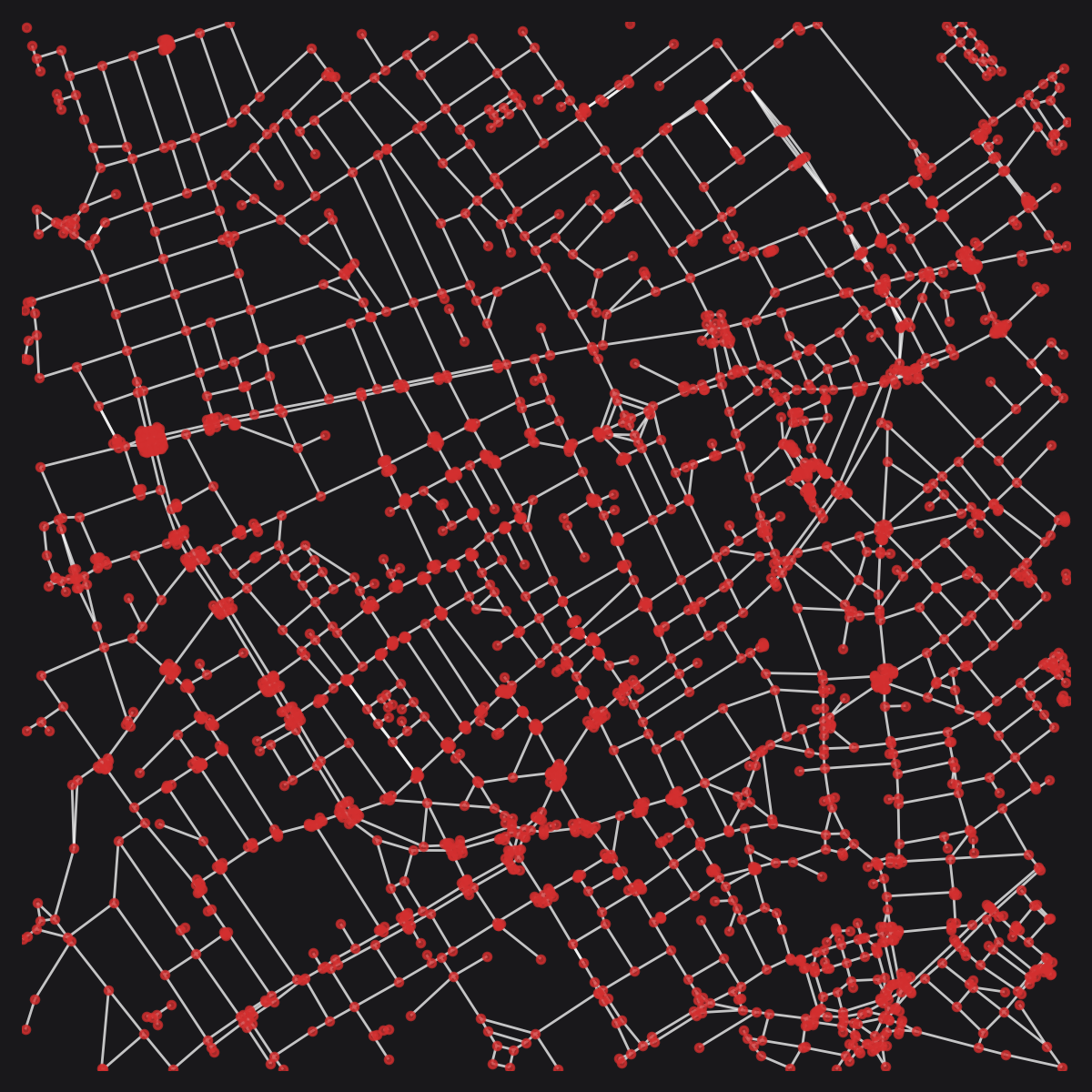The pre-consolidation OSM street network for Soho, London. © OpenStreetMap contributors.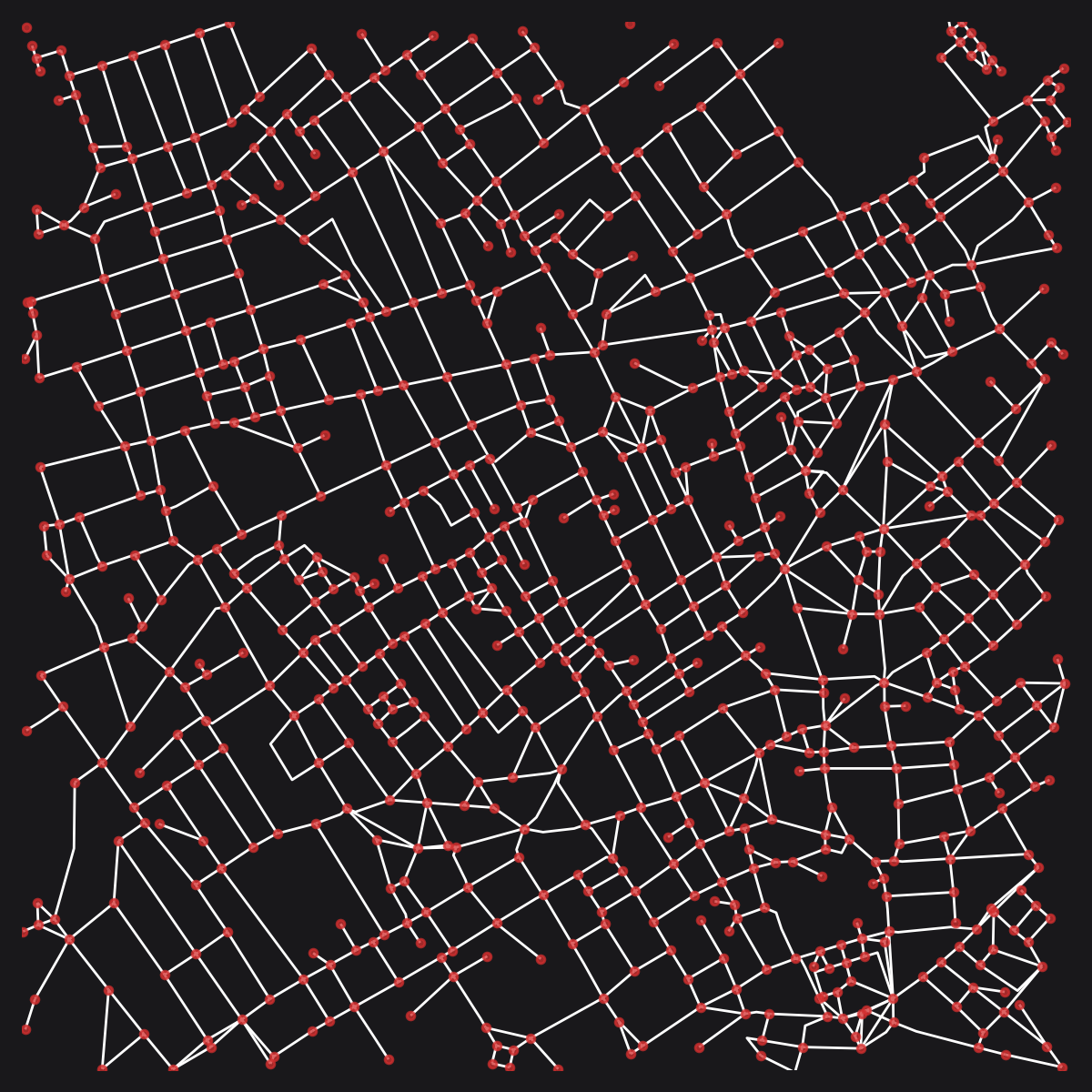The consolidated OSM street network for Soho, London. © OpenStreetMap contributors.

## nx_split_opposing_geoms

nx_split_opposing_geoms(
nx_multigraph,
buffer_dist=10,
merge_edges_by_midline=True,
contains_buffer_dist=20)

Split edges opposite nodes on parallel edge segments if within a buffer distance. This facilitates merging parallel roadways through subsequent use of nx-consolidate-nodes.

The merging of nodes can create parallel edges with mutually shared nodes on either side. These edges are replaced by a single new edge, with the new geometry selected from either:

• An imaginary centreline of the combined edges if merge_edges_by_midline is set to True;
• Else, the shortest edge, with longer edges discarded; See merge_parallel_edges for more information.

### Parameters

nx_multigraph
MultiGraph

A networkX MultiGraph in a projected coordinate system, containing x and y node attributes, and geom edge attributes containing LineString geoms.

buffer_dist
int

The buffer distance to be used for splitting nearby nodes. Defaults to 5.

merge_edges_by_midline
bool

Whether to merge parallel edges by an imaginary centreline. If set to False, then the shortest edge will be retained as the new geometry and the longer edges will be discarded. Defaults to True.

contains_buffer_dist
float

The buffer distance to consider when checking if parallel edges are sufficiently similar to be merged.

### Returns

MultiGraph

A networkX MultiGraph with consolidated nodes.

## nx_decompose

nx_decompose(
nx_multigraph,
decompose_max)

Decomposes a graph so that no edge is longer than a set maximum. Decomposition provides a more granular representation of potential variations along street lengths, while reducing network centrality side-effects that arise as a consequence of varied node densities.

Setting the decompose parameter too small in relation to the size of the graph may increase the computation time unnecessarily for subsequent analysis. For larger-scale urban analysis, it is generally not necessary to go smaller 20m, and 50m may already be sufficient for the majority of cases.

### Parameters

nx_multigraph
MultiGraph

A networkX MultiGraph in a projected coordinate system, containing x and y node attributes, and geom edge attributes containing LineString geoms.

decompose_max
float

The maximum length threshold for decomposed edges.

### Returns

MultiGraph

A decomposed networkX graph with no edge longer than the decompose_max parameter. If live node attributes were provided, then the live attribute for child-nodes will be set to True if either or both parent nodes were live. Otherwise, all nodes wil be set to live=True. The length and imp_factor edge attributes will be set to match the lengths of the new edges.

### Notes

from cityseer.tools import mock, graphs, plot

G = mock.mock_graph()
G_simple = graphs.nx_simple_geoms(G)
G_decomposed = graphs.nx_decompose(G_simple, 100)
plot.plot_nx(G_decomposed)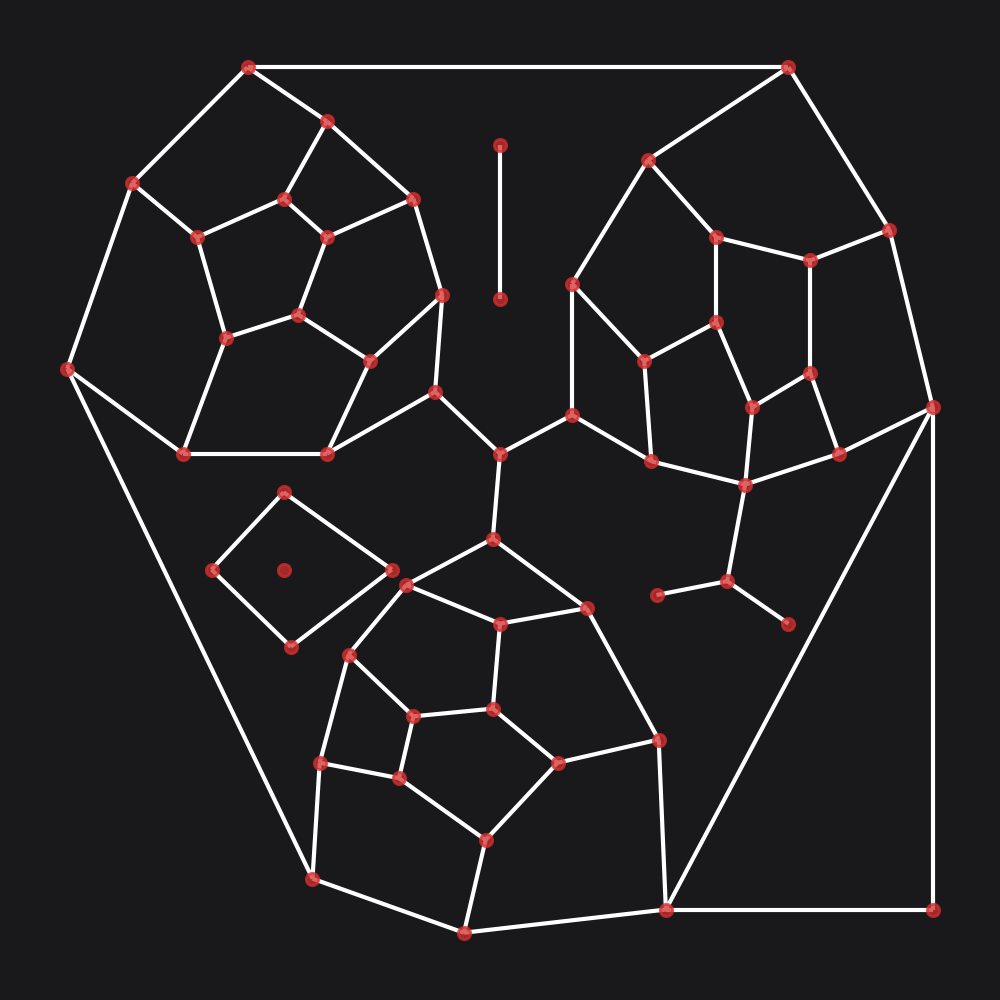Example graph prior to decomposition.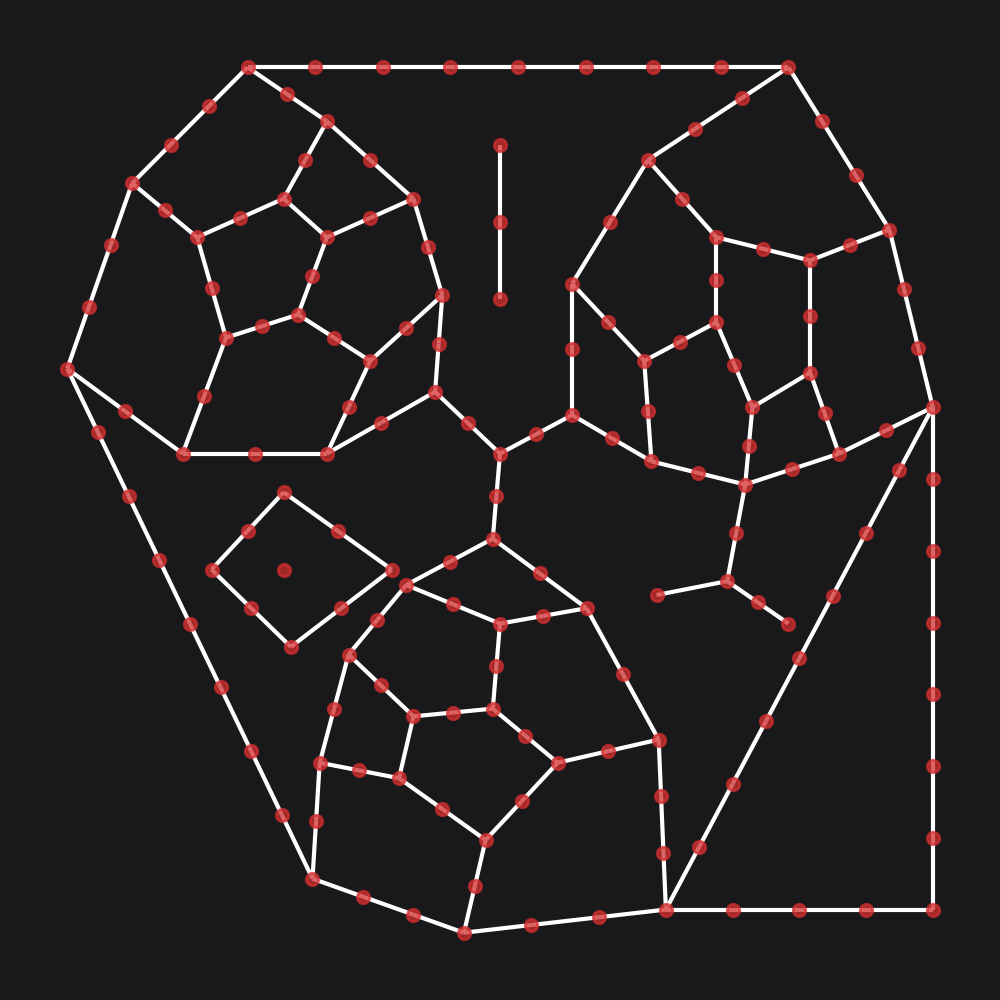Example graph after decomposition.

## nx_to_dual

nx_to_dual(
nx_multigraph)

Convert a primal graph representation to the dual representation. Primal graphs represent intersections as nodes and streets as edges. This method will invert this representation so that edges are converted to nodes and intersections become edges. Primal edge geom attributes will be welded to adjacent edges and split into the new dual edge geom attributes.

Note that a MultiGraph is useful for primal but not for dual, so the output MultiGraph will have single edges. e.g. a crescent street that spans the same intersections as parallel straight street requires multiple edges in primal. The same type of situation does not arise in the dual because the nodes map to distinct edges regardless.

### Parameters

nx_multigraph
MultiGraph

A networkX MultiGraph in a projected coordinate system, containing x and y node attributes, and geom edge attributes containing LineString geoms.

### Returns

MultiGraph

A dual representation networkX graph. The new dual nodes will have x and y node attributes corresponding to the mid-points of the original primal edges. If live node attributes were provided, then the live attribute for the new dual nodes will be set to True if either or both of the adjacent primal nodes were set to live=True. Otherwise, all dual nodes wil be set to live=True. The primal geom edge attributes will be split and welded to form the new dual geom edge attributes. A parent_primal_node edge attribute will be added, corresponding to the node identifier of the primal graph.

### Notes

from cityseer.tools import graphs, mock, plot

G = mock.mock_graph()
G_simple = graphs.nx_simple_geoms(G)
G_dual = graphs.nx_to_dual(G_simple)
plot.plot_nx_primal_or_dual(G_simple,
G_dual,
plot_geoms=False)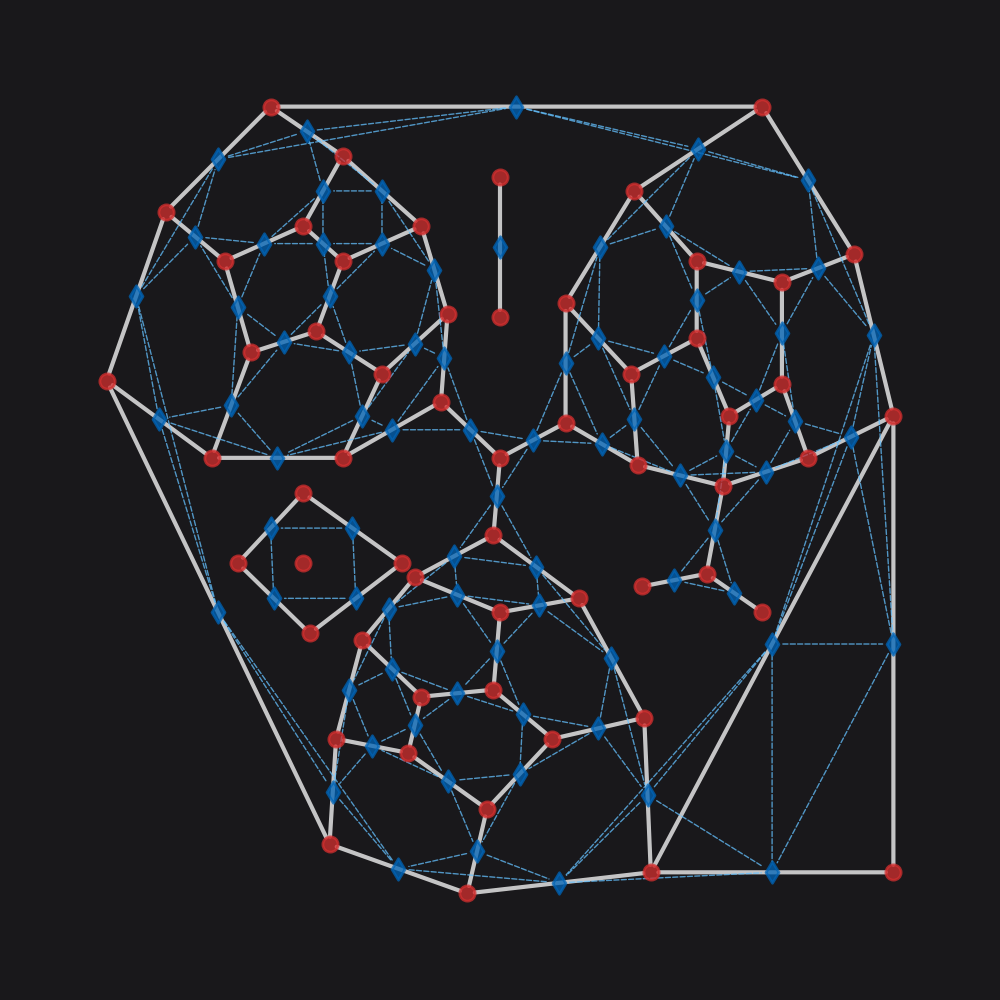Dual graph (blue) overlaid on the source primal graph (red).

## network_structure_from_nx

network_structure_from_nx(
nx_multigraph,
crs)

Transpose a networkX MultiGraph into a GeoDataFrame and NetworkStructure for use by cityseer. Calculates length and angle attributes, as well as in and out bearings, and stores this information in the returned data maps.

### Parameters

nx_multigraph
MultiGraph

A networkX MultiGraph in a projected coordinate system, containing x and y node attributes, and geom edge attributes containing LineString geoms.

crs
str | int

CRS for initialising the returned structures. This is used for initialising the GeoPandas GeoDataFrame. # pylint: disable=line-too-long

### Returns

nodes_gdf
GeoDataFrame

A GeoDataFrame with live and geometry attributes. The original networkX graph’s node keys will be used for the GeoDataFrame index.

network_structure
structures.NetworkStructure

## nx_from_network_structure

nx_from_network_structure(
nodes_gdf,
network_structure,
nx_multigraph)

Write cityseer data graph maps back to a networkX MultiGraph. This method will write back to an existing MultiGraph if an existing graph is provided as an argument to the nx_multigraph parameter.

### Parameters

nodes_gdf
GeoDataFrame

A GeoDataFrame with live and Point geometry attributes. The index will be used for the returned networkX graph’s node keys.

network_structure
structures.NetworkStructure

A structures.NetworkStructure instance corresponding to the nodes_gdf parameter.

nx_multigraph
MultiGraph

An optional networkX graph to use as a backbone for unpacking the data. The number of nodes and edges should correspond to the cityseer data maps and the node identifiers should correspond to the node_keys. If not provided, then a new networkX graph will be returned. This function is intended to be used for situations where cityseer data is being transposed back to a source networkX graph. Defaults to None.

### Returns

nx_multigraph
MultiGraph

A networkX graph. If a backbone graph was provided, a copy of the same graph will be returned. If no graph was provided, then a new graph will be generated. x, y, live node attributes will be copied from nodes_gdf to the graph nodes. length, angle_sum, imp_factor, in_bearing, and out_bearing attributes will be copied from the network_structure to the graph edges. cc_metric columns will be copied from the nodes_gdf GeoDataFrame to the corresponding nodes in the returned MultiGraph.